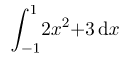## Welcome to the World of Modelling and Simulation

### What is Modelling?

This blog is all about system dynamics modelling and simulation applied in the engineering field, especially mechanical, electrical, and ...### Learning Mathematica, Lesson 3: Integration

Integration becomes very handy if you choose to use Wolfram Mathematica. You can evaluate definite and indefinite integrals using two ways.

1. You may use "Integrate[f, x]" command for the indefinite integrals, and "Integrate[f, {x, upper limit, lower limit}]" for the definite integrals.

2. Useintto enter  and then useto enter the lower limit, thenfor the upper limit:

For example, if you would like to find out the following integral of a function using both approaches,

Now, let's look at another example, where you will find detailed Mathematica codes to determine the integral of the following:Note that the following Mathematica program also implements a numerical integration scheme by using a built-in "NIntregate" command. Look carefully the systaxes used in the following codes to display the results, you may represent them in your own fashion.

Mathematica Codes:
(*Showing Exact Integration of First Problem*)
Print["Exact solution of \!\(\*SubsuperscriptBox[\(\[Integral]\), \
\(-1\), \(1\)]\)(2\!\(\*SuperscriptBox[\(x\), \(2\)]\)+3)\
\[DifferentialD]x"]
FullSimplify[\!\(
\*SubsuperscriptBox[\(\[Integral]\), \(-1\), \(1\)]\(\((2
\*SuperscriptBox[\(x\), \(2\)] + 3)\) \[DifferentialD]x\)\)]

(*Showing Numerical Integration of First Problem*)
Print["Numerical Integration by Approximation: \
\!\(\*SubsuperscriptBox[\(\[Integral]\), \(-1\), \
\(1\)]\)(2\!\(\*SuperscriptBox[\(x\), \(2\)]\)+3)\[DifferentialD]x"]
NIntegrate[(2 x^2 + 3), {x, -1, 1}]
Print["Numerical Integration by Gaussian Rule Order-1: \
\!\(\*SubsuperscriptBox[\(\[Integral]\), \(-1\), \
\(1\)]\)(2\!\(\*SuperscriptBox[\(x\), \(2\)]\)+3)\[DifferentialD]x"]
a = 2 x^2 + 3 /. x -> -Sqrt[1/(3)];
b = 2 x^2 + 3 /. x -> Sqrt[1/(3)];

c = N[a + b]

Program Output: